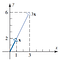# Eigenvalues and eigenvectors: a full information guide [LA4]

• Eigenvalues and Eigenvectors
• Diagonalization
• Calculating eigenvalue and eigenvector in R

# 1. The determinant of a matrix

We use determinants a lot in Linear Algebra, especially in the calculation of eigenvalues and eigenvectors. What is a determinant then?

## Properties of determinants

a) Scalar multiplication: if we multiply a column of a matrix by k the determinant is multiplied by k.

## a) 2x2 matrices:

Consider a 2x2 matrix

## b) 3x3 matrices:

Consider a 3x3 matrix

## c) n x n matrices:

We calculate the determinant of a n x n matrix A with

# 2. Eigenvalues and Eigenvectors

If A is a n x n matrix, then a non-null vector v in ℝ^n is called an eigenvector of A (or of the linear transformation T) if Av is a scalar multiple of v, that is: Av = λv
with some scalar λ. The scalar λ is called the eigenvalue of A (or of the linear transformation T), and we say that v is an eigenvector associated with λ.Matrix A expanded by a factor 3. Image from Anton & Rorren (2012).

# 3. Diagonalization

In this section, we will find a basis of ℝ^n that is based on the eigenvectors of a given matrix A n x n.

# 4. Calculating eigenvalues and eigenvectors in R

Calculate eigenvalues and eigenvectors in R is such an easy thing to do. First, we have to create a square matrix. You could use a correlation/covariance matrix, for example. But we will use a matrix from a previous example instead:

`A <- matrix(c(1/2, 0, 0, -1, 2/3, 0, 5, -8, -1/4), nrow = 3print(A)`
`##      [,1] [,2] [,3]## [1,]  1/2   0    0## [2,]  -1   2/3   0## [3,]   5   -8  -1/4`

Written by